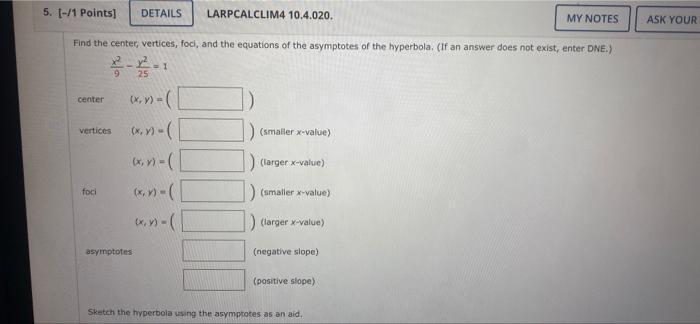Question:

# Find the center, vertices, foci, and the equations of the asymptotes of the hyperbola. (If an answer does not exist, enter DNE.) (x^2)/9 - (y)^2/25 =1 a)center (x/y) = ____ b)vertices (x/y)=____ (sFind the center, vertices, foci, and the equations of the asymptotes of the hyperbola. (If an answer does not exist, enter DNE.) (x^2)/9 - (y)^2/25 =1 a)center (x/y) = ____ b)vertices (x/y)=____ (smaller x-value) (x/y)=____(larger x-value) c)foci (x/y) =____(smaller x-value) (x/y) =____(larger x-value) d)asymptotes (x/y) =____ (smaller x-value) (x/y) =____(larger x-value)